# Title Changes in Circumference Problem Statement

 InterMath Title Changes in Circumference Problem Statement What happens to the circumference of a circle if you double the diameter? If you triple the diameter? If you halve the diameter? As the diameter increases (or decreases) in measure, how does the circumference change? Why does this change occur? Problem setup I start off with a circle then double the diameter. I go back to the original circle and triple the diameter. Each time the diameter is increased or decreased, I will measure the circumference. Plans to Solve/Investigate the Problem I plan to construct a circle using GSP. The circle will have a set of two radii that go through the center of the circle to create the diameter. One of the radii will be the radius. I will then find the circumference of the circle. To show the circumference change, I will then change the diameter to show how the circumference increases or decreases proportionally. Investigation/Exploration of the Problem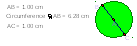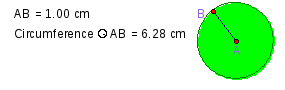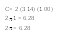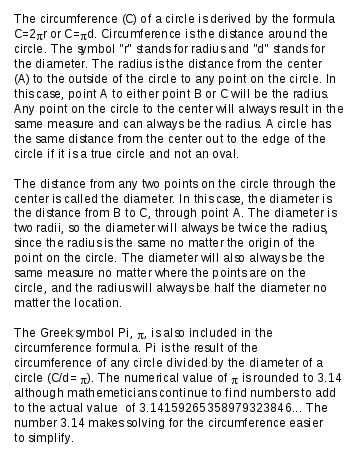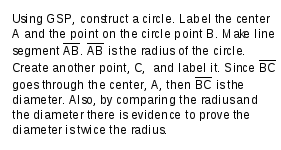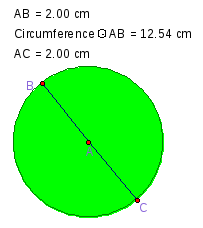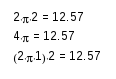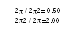B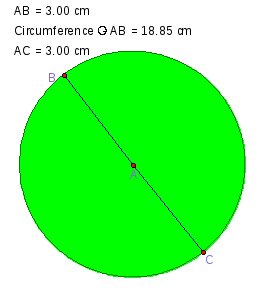y looking at the figure to the right and the ones above, there is obvious increase in size. The circumference is also increasing in certain increments. From the 1 cm diameter to the 2 cm, the circumference increases two times the original 1 cm. The diameter increases two times as well. From the 1 cm to the 3 cm, a diameter increase of three times, the circumference also increases three times. A circle with any size diameter that has an increase or decrease of a certain amount in measure will cause the same proportional increase or decrease in the circumference. Below is another example of how a diameter is increased to two and three times the original. When the diameter of 7 cm decreases by half, the circumference 43.99 cm decreases to half as well and becomes 22.01 cm. The measurements are off slightly in measuring exactly half because of pi. Without rounding pi to 3.14 the circumferences are exactly half. Measurements are often off by a tens or hundred place.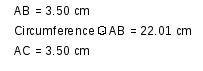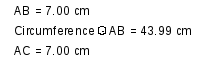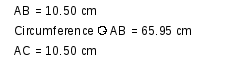The increasing increments result because of the constant pi. Since there is a constant, 3.14, any increase in the diameter, from an original circle, will result as the same amount increase in the circumference. Pi plays a significant role in having a proportional increase. For any circle the same principles will hold. Any increase in diameter or circumference by a certain measure or multiple causes the same change in the other. They increase or decrease proportionally or in the same increments. Share with your friends: